# Order parameters

An order parameter is some observable physical quantity that is able to distinguish between two distinct phases. The choice of order parameter is not necessarily unique.

## Solid-liquid transition

Possible choices:

• Fourier transform of the density
• Shear modulus

## Isotropic-nematic transition

The uniaxial order parameter is zero for an isotropic fluid and one for a perfectly aligned system. First one calculates a director vector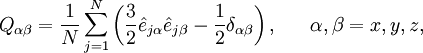$Q_{\alpha \beta} = \frac{1}{N} \sum_{j=1}^{N} \left( \frac{3}{2} \hat e_{j \alpha} \hat e_{j \beta} -\frac{1}{2} \delta_{\alpha\beta}\right),~~~~~\alpha, \beta = x, y, z,$

where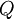$Q$ is a second rank tensor,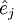$\hat e_{j}$ is a unit vector along the molecular long axis, and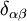$\delta_{\alpha\beta}$ is the Kronecker delta. Diagonalisation of this tensor gives three eigenvalues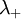$\lambda_+$,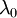$\lambda_0$ and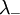$\lambda_-$, and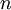$n$ is the eigenvector associated with the largest eigenvalue ($\lambda_+$). From this director vector the nematic order parameter is calculated from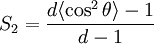$S_2 =\frac{d \langle \cos^2 \theta \rangle -1}{d-1}$

where d is the dimensionality of the system.

i.e. in three dimensions$S_2 = \lambda _{+}= \langle P_2( n \cdot e)\rangle = \langle P_2(\cos\theta )\rangle =\langle \frac{3}{2} \cos^{2} \theta - \frac{1}{2} \rangle$

where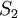$S_2$ is known as the uniaxial order parameter. Here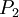$P_2$ is the second order Legendre polynomial,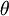$\theta$ is the angle between a molecular axes and the director$n$, and the angle brackets indicate an ensemble average.